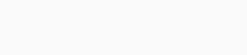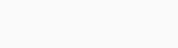# Check if a string is lapindrome or not in Python

Hello Coders, this tutorial deals with a program to check whether a string is lapindrome or not in Python. Also, we can say that we will check if the strings have same set of characters or not in Python.
Let’s start.

## Lapindrome

Strings when divided into halves then if the partitioned strings have the same frequency(i.e character count) on both the partitions then those strings are called lapindromes. If there are odd no. of characters then ignore middle character.

Example:

abcdcba – this is a lapindrome as if we split it into two halves then we get “abc” and “cba”. We can see that characters a,b,c have the same frequency on both the halves.

abba – this is a lapindrome as if we split it into two halves then we get “ab” and “ba”. We can see that characters a,b have the same frequency on both the halves.

### Algorithm:

1. Taking input in a variable named “s”.
2.  Partitioning s into two halves s1,s2 (if odd no. of characters then to ignore the middle character).
3.  Storing in a list named l1, l2 and sorting them in ascending order.
4.  Converting it into the string and checking if the two halves are the same or not.

### Python program to check if a string is lapindrome or not

```s=input()
s1,s2='',''
if(len(s)%2==0):
s1=s[:len(s)//2]
s2=s[len(s)//2:]
else:
s1=s[:len(s)//2]
s2=s[len(s)//2+1:]
l1=list(s1)
l2=list(s2)
l1.sort()
l2.sort()
s1=str(l1)
s2=str(l2)
if(s1==s2):
print('YES')
else:
print('NO')```

Output 1:Output 2:For any more queries please comment below.